/scikit-learn

# sklearn.model_selection.StratifiedKFold

`class sklearn.model_selection.StratifiedKFold(n_splits=’warn’, shuffle=False, random_state=None)` [source]

Stratified K-Folds cross-validator

Provides train/test indices to split data in train/test sets.

This cross-validation object is a variation of KFold that returns stratified folds. The folds are made by preserving the percentage of samples for each class.

Read more in the User Guide.

Parameters: `n_splits : int, default=3` Number of folds. Must be at least 2. Changed in version 0.20: `n_splits` default value will change from 3 to 5 in v0.22. `shuffle : boolean, optional` Whether to shuffle each stratification of the data before splitting into batches. `random_state : int, RandomState instance or None, optional, default=None` If int, random_state is the seed used by the random number generator; If RandomState instance, random_state is the random number generator; If None, the random number generator is the RandomState instance used by `np.random`. Used when `shuffle` == True.

`RepeatedStratifiedKFold`
Repeats Stratified K-Fold n times.

#### Notes

Train and test sizes may be different in each fold, with a difference of at most `n_classes`.

#### Examples

```>>> from sklearn.model_selection import StratifiedKFold
>>> X = np.array([[1, 2], [3, 4], [1, 2], [3, 4]])
>>> y = np.array([0, 0, 1, 1])
>>> skf = StratifiedKFold(n_splits=2)
>>> skf.get_n_splits(X, y)
2
>>> print(skf)
StratifiedKFold(n_splits=2, random_state=None, shuffle=False)
>>> for train_index, test_index in skf.split(X, y):
...    print("TRAIN:", train_index, "TEST:", test_index)
...    X_train, X_test = X[train_index], X[test_index]
...    y_train, y_test = y[train_index], y[test_index]
TRAIN: [1 3] TEST: [0 2]
TRAIN: [0 2] TEST: [1 3]
```

#### Methods

 `get_n_splits`([X, y, groups]) Returns the number of splitting iterations in the cross-validator `split`(X, y[, groups]) Generate indices to split data into training and test set.
`__init__(n_splits=’warn’, shuffle=False, random_state=None)` [source]
`get_n_splits(X=None, y=None, groups=None)` [source]

Returns the number of splitting iterations in the cross-validator

Parameters: `X : object` Always ignored, exists for compatibility. `y : object` Always ignored, exists for compatibility. `groups : object` Always ignored, exists for compatibility. `n_splits : int` Returns the number of splitting iterations in the cross-validator.
`split(X, y, groups=None)` [source]

Generate indices to split data into training and test set.

Parameters: `X : array-like, shape (n_samples, n_features)` Training data, where n_samples is the number of samples and n_features is the number of features. Note that providing `y` is sufficient to generate the splits and hence `np.zeros(n_samples)` may be used as a placeholder for `X` instead of actual training data. `y : array-like, shape (n_samples,)` The target variable for supervised learning problems. Stratification is done based on the y labels. `groups : object` Always ignored, exists for compatibility. `train : ndarray` The training set indices for that split. `test : ndarray` The testing set indices for that split.

#### Notes

Randomized CV splitters may return different results for each call of split. You can make the results identical by setting `random_state` to an integer.

## Examples using `sklearn.model_selection.StratifiedKFold`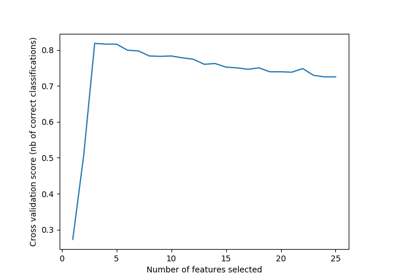Recursive feature elimination with cross-validation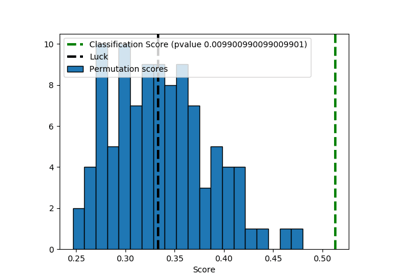Test with permutations the significance of a classification score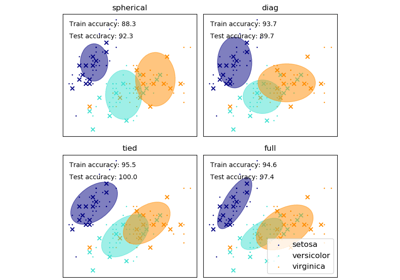GMM covariances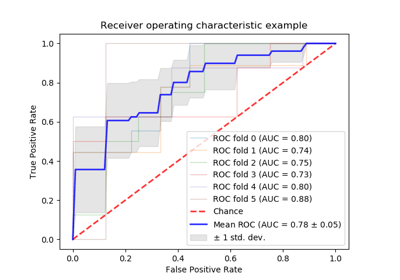Receiver Operating Characteristic (ROC) with cross validation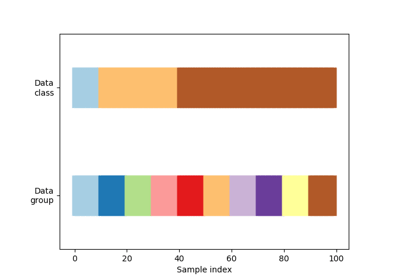Visualizing cross-validation behavior in scikit-learn